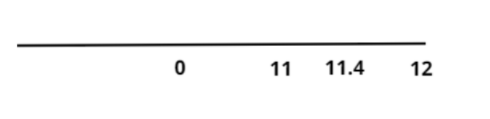Courses
Courses for Kids
Free study material
Free LIVE classes
MoreLIVE
Join Vedantu’s FREE Mastercalss

# Round the decimal number to the nearest one.11.4Verified
361.5k+ views
Hint: Round off is the process of replacing the given number that is to be round off with an approximate value which is more precise, shorter or say simpler in comparison to the given number.
Let’s talk about plotting the given number of 11.4 onto a number line.Now it is clear from this number line that the closest integral value to 11.4 is 11 only. 12 is another value which can be doubted to be closer to 11.4 so let’s check for 12.
$12 - 11.4 = 0.6$…………………. (1)
Now let’s check for 11.
$11.4 - 11 = 0.4$…………………. (2)
Clearly from equation (1) and equation (2) we can say that $0.4 < 0.6$or 11 is closer to 11.4 in comparison to 12.
Thus the round off for 11.4 will be 11.

Note: Whenever we face such types of problems the key concept is simply to plot the given number onto a number line, this will help getting the neighbors of that number that are doubtful to be closer to the required number. Then simply calculate how far that neighbor is with respect to the given number is. The least far neighbor which is also an integer will give the right answer.
Last updated date: 20th Sep 2023
Total views: 361.5k
Views today: 3.61k